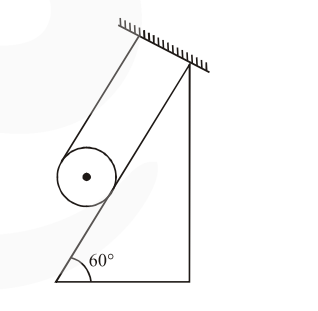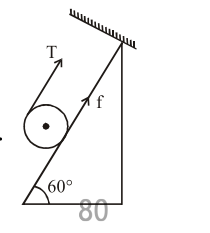# A solid cylinder of mass m is wrapped`
Question:

A solid cylinder of mass $m$ is wrapped with an inextensible light string and, is placed on a rough inclined plane as shown in the figure. The frictional force acting between the cylinder and the inclined plane is :[The coefficient of static friction, $\mu_{\mathrm{s}}$, is $\left.0.4\right]$

1. $\frac{7}{2} \mathrm{mg}$

2. $5 \mathrm{mg}$

3. $\frac{\mathrm{mg}}{5}$

4. 0

Correct Option: , 3

Solution:Let's take solid cylinder is in equilibrium

$T+f=m g \sin 60$        ...................(1)

$\mathrm{TR}-\mathrm{fR}=0$       ................(2)

Solving we get

$\mathrm{T}=\mathrm{f}_{\mathrm{req}}=\frac{\mathrm{mg} \sin \theta}{2}$

But limiting friction $<$ required friction

$\mu \mathrm{mg} \cos 60^{\circ}<\frac{\mathrm{mg} \sin 60^{\circ}}{2}$

$\therefore$ Hence cylinder will not remain in equilibrium

Hence $f=$ kinetic

$=\mu_{\mathrm{k}} \mathrm{N}$

$=\mu_{\mathrm{k}} m g \cos 60^{\circ}$

$=\frac{m g}{5}$